Ex 13.2

Chapter 13 Class 8 Introduction to Graphs
Serial order wise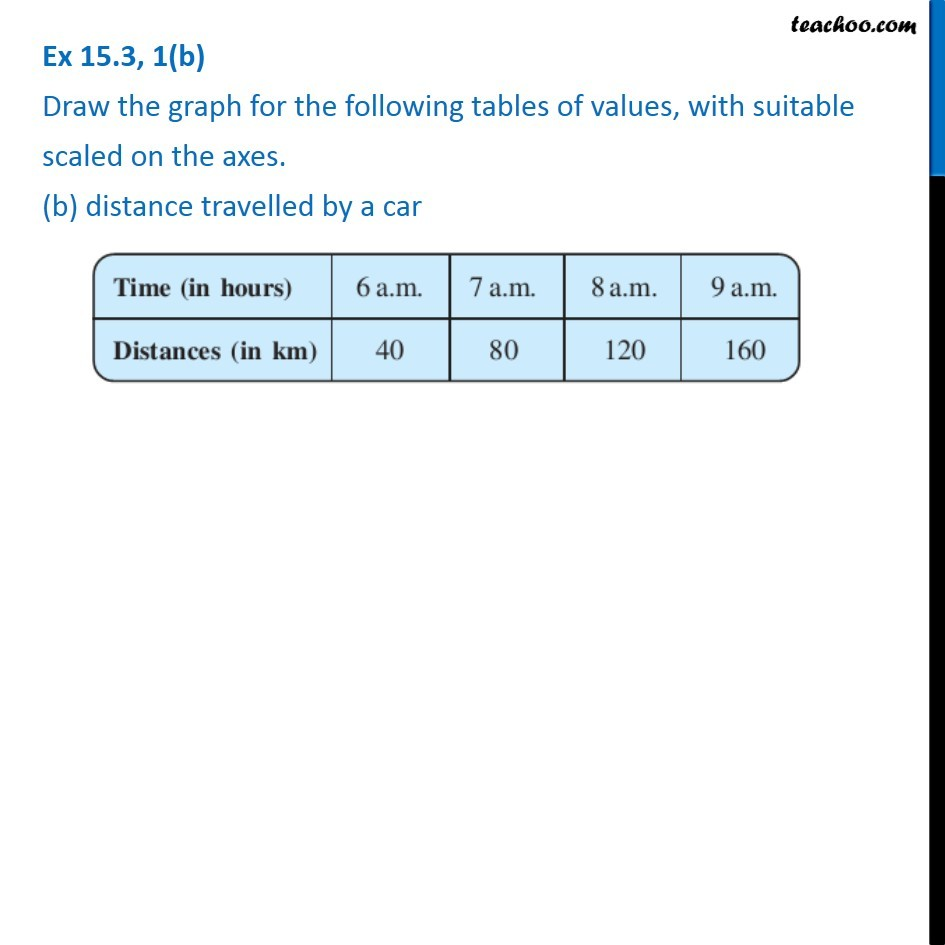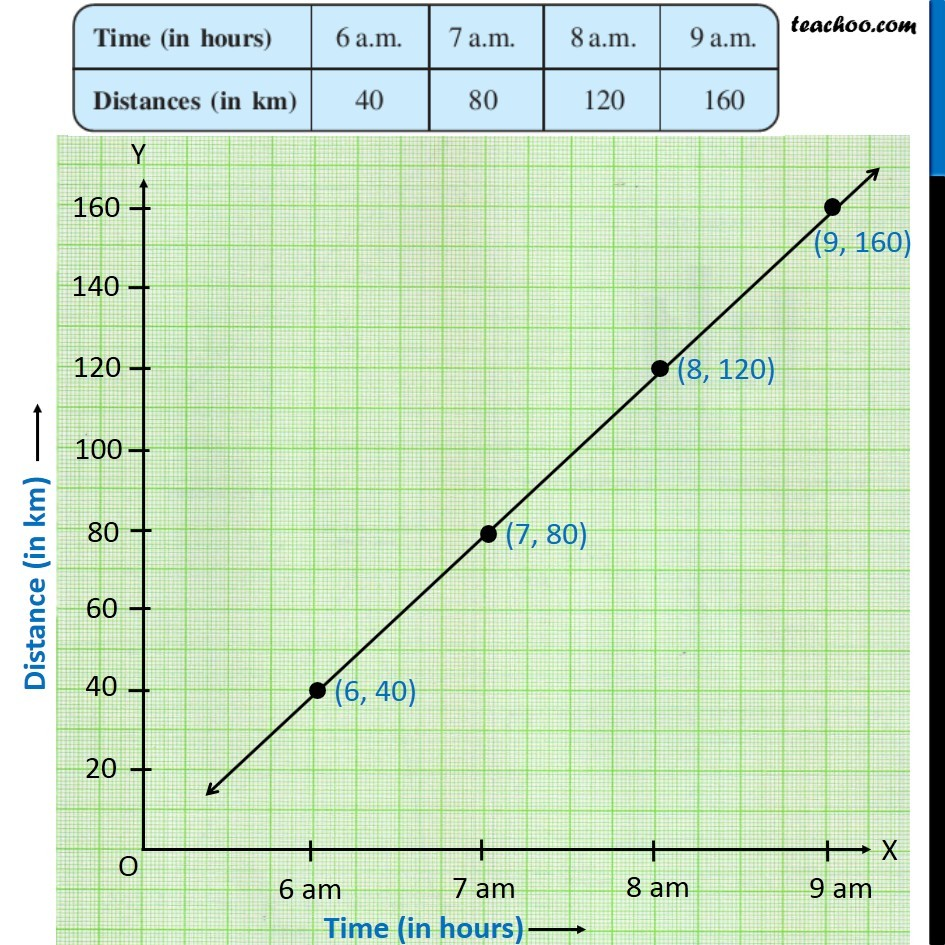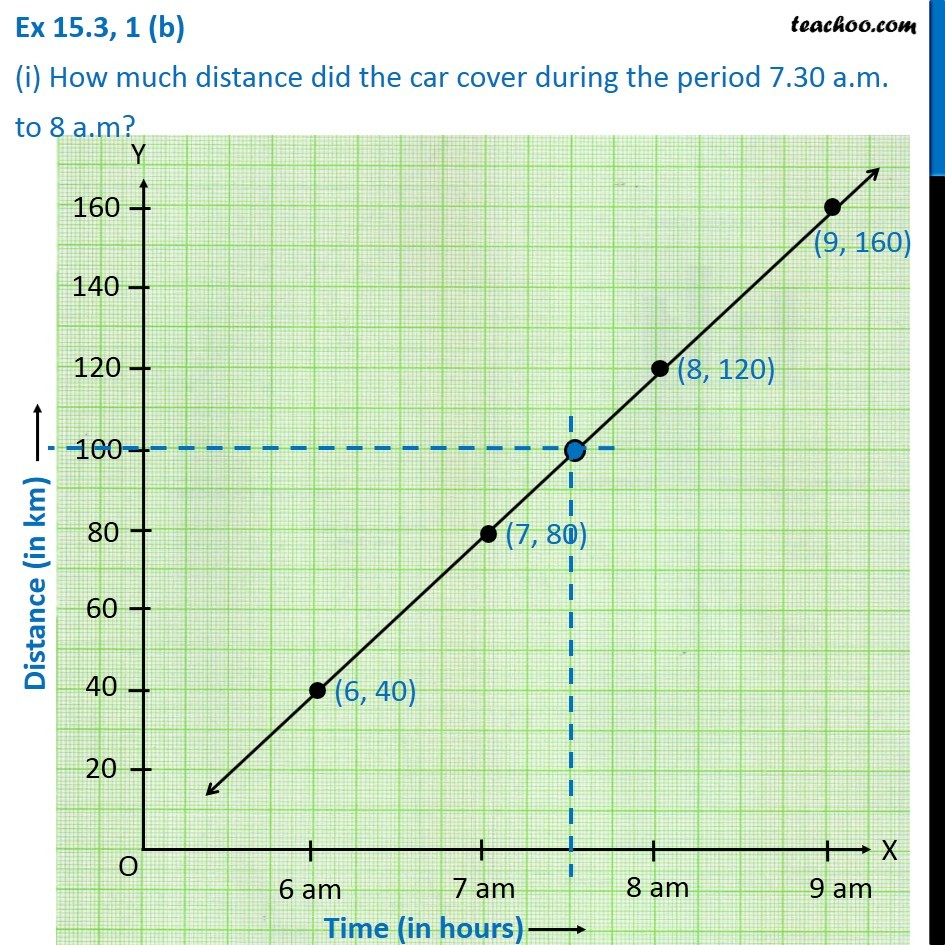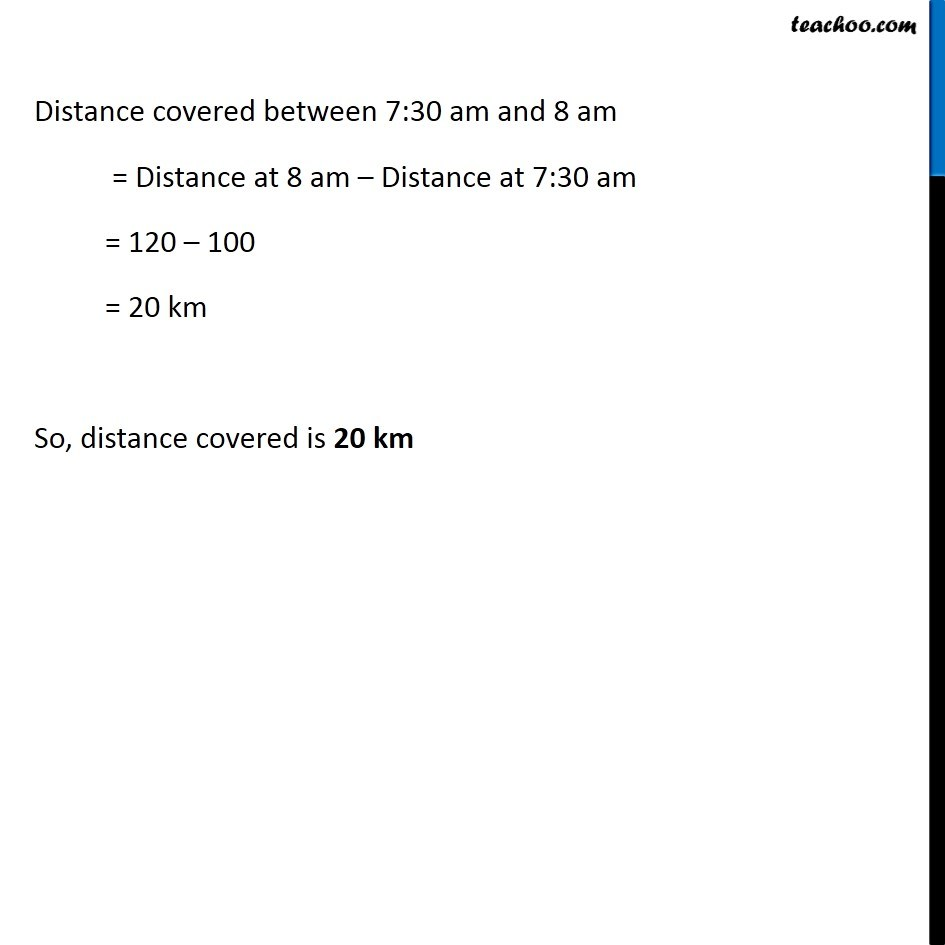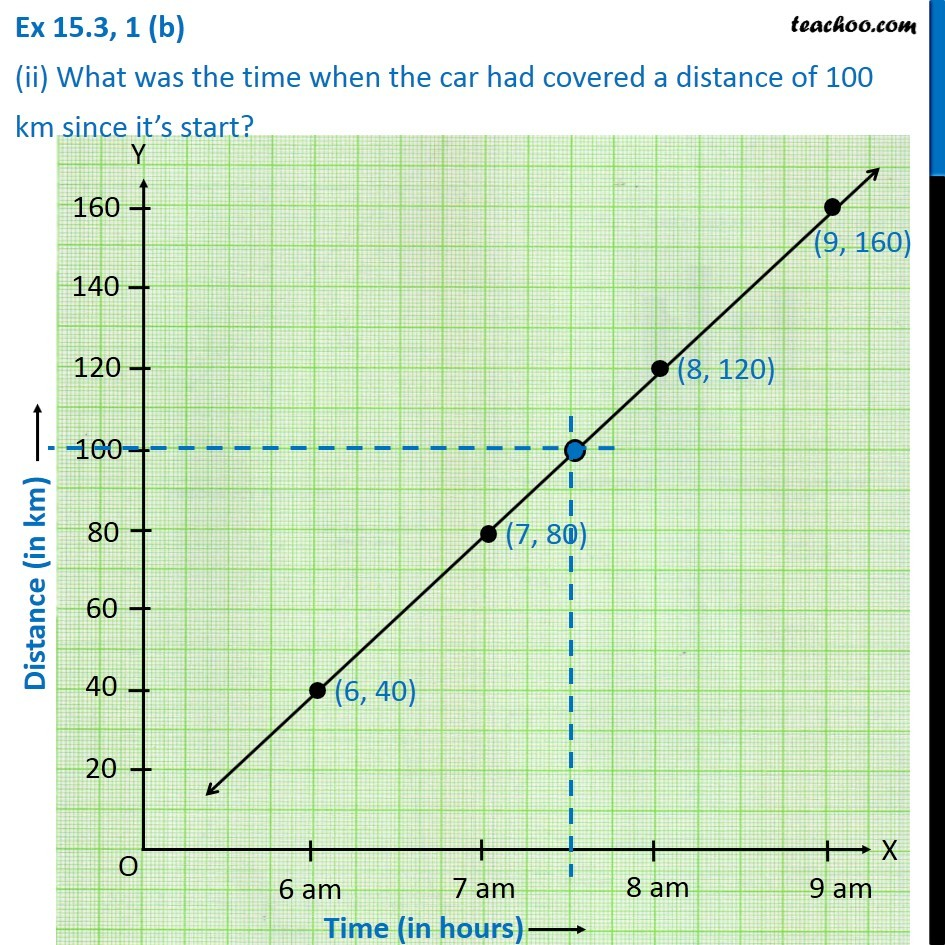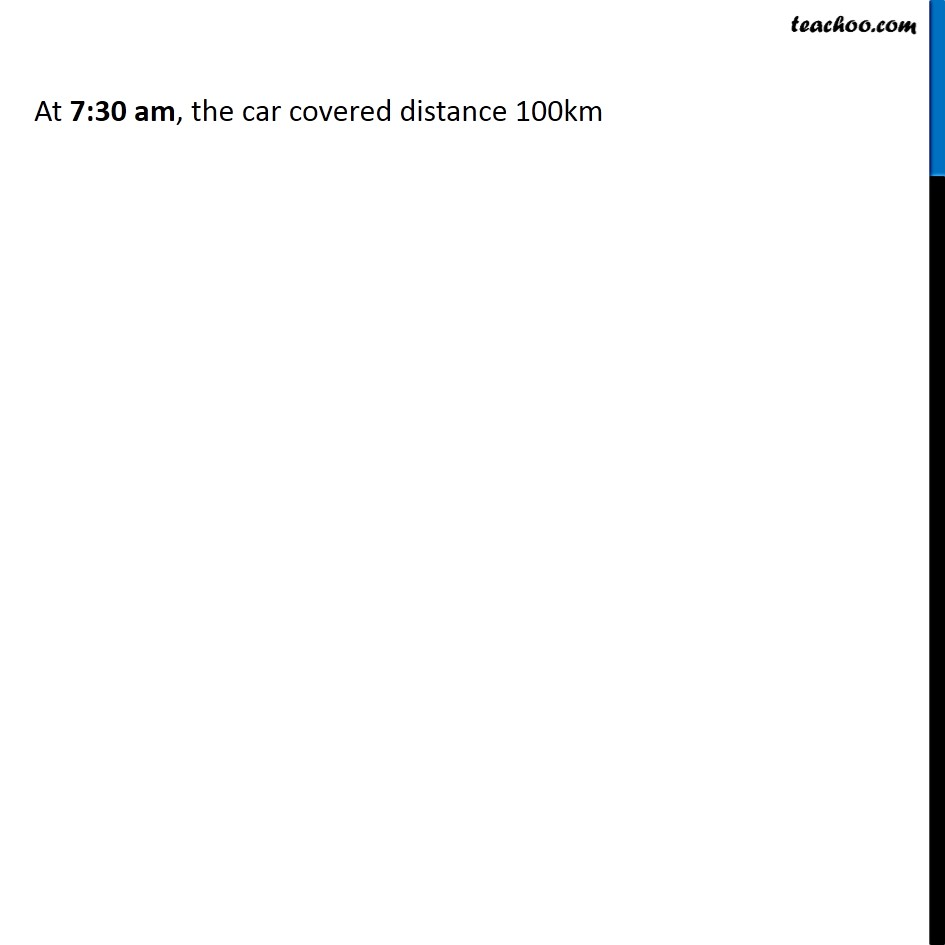Learn in your speed, with individual attention - Teachoo Maths 1-on-1 Class

### Transcript

Ex 13.2, 1(b) Draw the graph for the following tables of values, with suitable scaled on the axes. (b) distance travelled by a car Time (in hours) | 6 am | 7 am | 8 am | 9 am Distance (in km) | 40 | 80 | 120 | 160 Plotting Distance (in km), from 0 to 160 (with a gap of 20) and Time (in hours) in y-axis, from 0 to 9 am (with a gap of 5) Ex 13.2, 1 (b) (i) How much distance did the car cover during the period 7.30 a.m. to 8 a.m? Distance covered between 7:30 am and 8 am = Distance at 8 am – Distance at 7:30 am = 120 – 100 = 20 km So, distance covered is 20 km Ex 13.2, 1 (b) (ii) What was the time when the car had covered a distance of 100 km since it’s start? Drawing a vertical line from 7:30 am, Where it intersects the line, draw a horizontal line, distance is 100 km At 7:30 am, the car covered distance 100km